# Functional Principal Component Analysis with Spark

## 1.) Functional Principal Component Analysis

Letbe a centered smooth random function in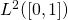, with finite second moment. Without loss of generality we assumeinstead of some arbitrary compact interval and only consider centered functions. If non-centered curves are assume than the curves can be centered by subtracting.

The underlying dependence structure can be characterized by the covariance function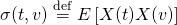and
the corresponding covariance operator(1)Letdenote the ordered eigenvalues ofand letbe a corresponding system of orthonormal
eigenfunctions (functional principal components) s.t.. The Karhunen-Loeve decomposition states that the random functionscan be represented in the form

(2)where the loadingsare random variables defined asthat satisfy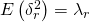, as well as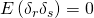for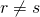.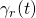are denoted as functional principal components andthe corresponding principal scores.

### 1.1) Example: Brownian motion

A nice analytical example where we can actually calculate the principal components is the brownian motion. Letbe a brownian motion on, then its probability density function is given by. Accordingly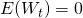and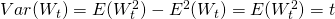. The covariance function withis then given bysince for,andare independent random variables. Thusand we have to solve the following eigenvalue problemwich can be rewritten as. Differentiating once leads to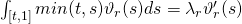this gives us two boundary conditions given byand. Differentiating again leads toIn fact this is the standart example from basic math curses introducing Sturm-Liouville and a solution is given by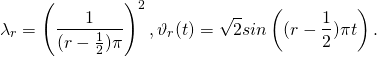Using thatwiththe Karhunen-Loeve decomposition is given by## 2.) A Spark implementation

Usually a sample ofcurves is observed, in addition Spark is not able to handle functions but is only able to process discrete data. To model these issues, letbe an i.i.d. sample of smooth curves with continuous covariance function, we assume that each curve in the sample is observed at an equidistant grid. We will thus use empirical approximation of the covariance function is then given by the sample covariance matrixto derive an estimator for the functional components one will usually rely on an eigenvalue decomposition of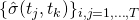. For the Spark implementation, let us focus now on an alternative way to estimate the Karhunen-Loeve decomposition based on the duality relation between row and column space. Let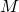be the dual matrix consisting of entries

(3)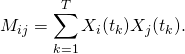The eigenvectors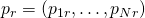and eigenvalues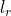of the matrixare connected to the empirical,and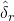, resulting in replacing the expectation inwith the empirical counterpart and the integral in (1) by a rieman sum, by,and### 2.1) Test the Algorithm using the Brownian MotionThe figure shows the first two true principal components of a brownian motion and the estimates from the presented algorithm

Let, we simulate a standard Brownian Motion at points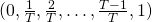withby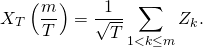Using Donsker’s theorem one can then show that for,converges in distribution to a standard brownian motion. To test the algorithm we samplecurves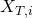atequidistant timepoints.

### 2.2) Implementation

As an primary example and to check if our algorithm works the way we intended, we will sample a set ofcurves from the space of brownian motions. These curves where computed at the master and then be transfered to the spark cluster where the Spark-FPCA is performed. The functional principal components  are then transferred back to the master where we compare our estimates with the true functional principal components  derived in the previous sections.

#We start with a sample of brownian motions
import matplotlib.pyplot as plt
from numpy import *
from pyspark.mllib.linalg import *
from pyspark.mllib.linalg.distributed import *
from pyspark.mllib.linalg.distributed import CoordinateMatrix, MatrixEntry

def spark_FPCA( matrix , L):
N = matrix.numRows()
T = matrix.numCols()
scale =CoordinateMatrix(sc.parallelize(range(N)).map(lambda i: MatrixEntry(i, i, 1./sqrt(T) )), N, N).toBlockMatrix()
# Compute the top L singular values and corresponding singular vectors.
svd = scale.multiply(matrix).transpose().toIndexedRowMatrix().computeSVD(L)
s = svd.s       # The singular values are stored in a local dense vector.
Va=svd.V.toArray()
Vl = list();
for i in range(0, len(Va)):
Vl.append( IndexedRow(i, Va[i] ))
V=IndexedRowMatrix( sc.parallelize(Vl) )
S=DenseMatrix( L,L, diag(s).flatten().flatten().tolist() )
Si=DenseMatrix( L,L, diag(1./(s)).flatten().flatten().tolist() )
scores=V.multiply(S)
components= matrix.transpose().multiply( V.multiply(Si).toBlockMatrix() )
#reduced FPCA decomposition
FPCA= components.multiply(scores.toBlockMatrix().transpose() )
return (components, scores, FPCA, s)

def reuse_FPCA( matrix , components):
N = matrix.numRows()
T = matrix.numCols()
scaler=CoordinateMatrix(sc.range(N).map(lambda i: MatrixEntry(i, i, 1./T)), N, N).toBlockMatrix()
scores=scaler.multiply(matrix).multiply( components )
FPCA= components.multiply(scores.transpose() )
return (components, scores.toIndexedRowMatrix(), FPCA)

##EXAMPLE USING A BROWNIAN MOTION

T=10000
N=400
L=2

data = list();
for i in range(0, N):
data.append( IndexedRow(i, array( cumsum(random.normal(0,sqrt(true_divide(1,T)),T)) ) ) )

#convert the data to spark
sc_data= sc.parallelize( data )
matrix = IndexedRowMatrix(sc_data).toBlockMatrix().cache()

result=spark_FPCA(matrix, 3)

FPCA=result.toLocalMatrix()
comp=result.toLocalMatrix()
scores_old=result.rows.collect()

#Component plots
times=true_divide( arange(0,T),T )
true_comp=list();
for i in range(1, L+1):
true_comp.append( sin( (i-0.5)*pi*times) )

for i in range(0,len(true_comp)):
plt.plot(times, true_comp[i])

for i in range(1,len(comp.toArray()[1,:])+1):
plt.plot(times, 0.7*comp.toArray()[:,(i-1)]) ##I have no idea where the scaling diff comes from... :(

plt.show()

##Construct new Brownian motion to reuse principal components
data = list();
for i in range(0, N):
data.append( IndexedRow(i, array( cumsum(random.normal(0,sqrt(true_divide(1,T)),T)) ) ) )

plt.plot(times, array( cumsum(random.normal(0,sqrt(true_divide(1,T)),T)) ) )
plt.show()

#convert the data to spark
sc_data= sc.parallelize( data )
matrix2 = IndexedRowMatrix(sc_data).toBlockMatrix().cache()
result2=reuse_FPCA(matrix2, result)

##New Scores
result2.rows.collect()


### 2 thoughts on “Functional Principal Component Analysis with Spark”

1.